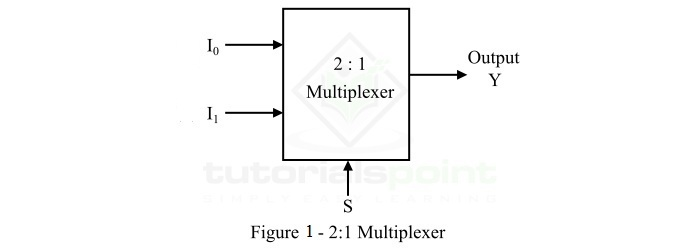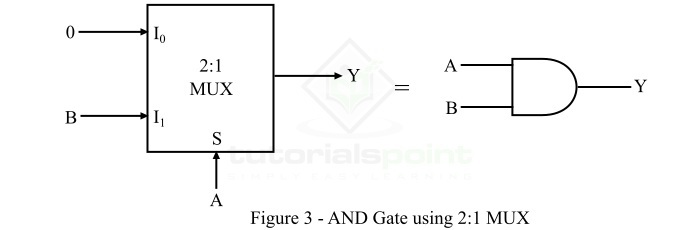# Realizing an AND Gate Using a 2:1 MUX

A multiplexer or MUX is a combinational circuit that accepts several data inputs and allows only one of them to flow through the output line. Multiplexer (MUX) is also known as data selector because it selects one from many.

A MUX consists of 2n data input lines, n select lines, and 1 output line. Since, it converts 2n input lines into 1 output line. Therefore, it is also called many-to-one device.

Depending upon the number of input lines, there are several types of multiplexer present such as 2:1 MUX, 4:1 MUX, 8:1 MUX, etc.

## What is 2:1 Multiplexer (MUX)?

The functional block diagram of a 2:1 MUX is shown in Figure-1.A 2:1 MUX consists of 2 (21) data input lines designated by I0 and I1, 1 select line designated by S and 1 output line Y. The logic level either 0 or 1 applied to the select line S determines which input data will pass through the output line of the multiplexer.

The operation of the 2:1 MUX can be analyzed with the help of its truth table shown below.

Select Line (S) Output (Y)
0 I0
1 I1

From this truth table, we can conclude that,

• If select line S is connected to logic level 0, the data input connected to I0 will pass through the output line Y.

• If select line S is connected to logic level 1, the data input connected to I1 will pass through the output line Y.

Now, let us discuss the basics of AND gate.

## What is an AND Gate?

AND Gate is a basic logic gate which may have two or more inputs, but only one output. The AND gate gives logic 0 state (LOW) as output if any one of its inputs is in the logic 0 state, otherwise, it gives a logic 1 states (HIGH) as output. Therefore, the output of the AND gate is HIGH or logic 1 state, only if all its inputs are HIGH or logic 1 state. The logic symbol of a two input AND gate is shown in Figure-2.The logic expression of the two input AND gate is given by,

$$Y=A\cdot B$$

Where, the ‘.’ (dot) symbol represents the AND operation. It is read as “Y is equal to A AND B”.

The operation of the AND gate can be understood with the help of its function table which is given below.

Inputs Output
ABY = A.B
000
010
100
111

After getting insights on basics of AND gate and 2:1 MUX, we are able to realize an AND gate with the help a 2:1 MUX which is described in the following section.

## Realizing an AND Gate by using a 2:1 Multiplexer

The functional block diagram of a 2:1 multiplexer equivalent to the AND gate is shown in Figure-3.Here, the input line I0 of the 2:1 MUX is set to logic 0 state. The input B of the AND gate is applied to the input line I1 of MUX. The input variable A of the AND gate is used to control select line of MUX.

The operation of the 2:1 MUX as the AND gate can be described as follows −

• When A = 0, the output of the MUX as AND gate is 0.

• When A = 1, the output of the MUX as AND gate is equal to B.

The function table of the 2:1 MUX working as an AND gate is given as follows,

A (S) B Y Description
0 0 0

Y = 0

When A = 0

1 0 0
1 0 0

Y = B

When A = 1

1 1 1

Hence, we can analyze it as follows,

$$Y=\bar{A}\cdot \bar{B}\cdot 0+ \bar{A}\cdot B \cdot 0+A\cdot \bar{B} \cdot 0+ A \cdot B \cdot 1=AB$$

In this way, we can implement the AND gate by using a 2:1 multiplexer.

Updated on: 19-Apr-2023

3K+ Views